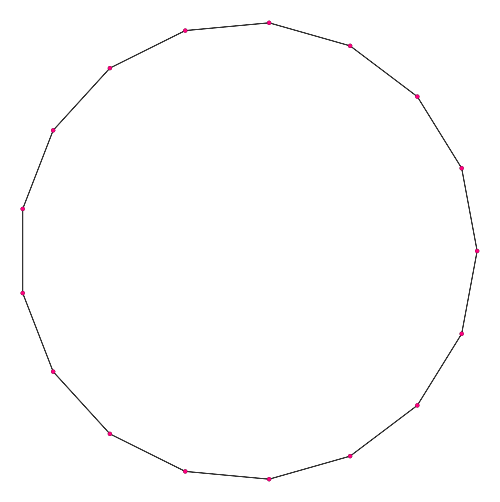# Origami

Firstly, I’ll take this opportunity to wish James Cranch a marvellous 30th birthday.

This post was inspired by the conception of a new ‘origami society’ at Trinity. Having never tried it before, it took me a while to decipher the instructions for the construction of a dragon.There are rather restrictive constraints on what possible patterns of folds can be realised. Firstly, arrangements of folds are represented as plane graphs (known as ‘crease patterns’) with two distinct types of edges, namely ‘mountain folds’ and ‘valley folds’. There is actually a third type of edge, a ‘boundary edge’, since we’re dealing with a finite square rather than R^2; the boundary edges form a cycle such that all other vertices lie in the closed subset of the plane bounded by this cycle.

• Maekawa’s theorem: Each non-boundary vertex must have even degree 2n, such that there are either n + 1 valley folds and n − 1 mountain folds, or vice-versa. As a consequence of the even degree property, the dual graph (or, more pedantically, the induced subgraph of the dual graph obtained by removing the vertex at infinity) has a chromatic number of 2.

This is the only combinatorial restriction, and yields graphs such as this one:There are, however, more geometrical constraints.

• Kawasaki’s theorem: the sum of the alternate angles around each interior vertex is π. This is a well-defined concept, since Maekawa’s theorem forces the degree of each interior vertex to be even. Also, the configuration must be realisable in Euclidean geometry.

Finally, there is the constraint that the three-dimensional embedding of the folded paper does not self-intersect. This is much more difficult to verify from the diagram than the other two properties.

### Postulates

Since origami requires precision, it would be ideal to be able to produce exact crease patterns. This can be accomplished with a pencil, compass and straightedge, as in Euclidean constructions. Basically, the operations achievable with compass and straightedge are:

1. Given two points, A and B, construct the unique line passing through A and B;
2. Given two points, A and B, construct the unique circle centred on A and passing through B;
3. Given two curves which intersect in finitely many points, construct these points of intersection.

It’s not too difficult to show that these operations are equivalent to solving a quadratic given its coefficients. Three famous classical problems, namely ‘doubling the cube’, ‘trisecting the angle’ and ‘squaring the circle’, are unsolvable with these operations (proof: Galois theory for the first two, and transcendentality of pi for the third).

It can be shown via Galois theory that the nth roots of unity can be constructed if and only if n is a product of a power of two and distinct primes of the form 2^k + 1 (i.e. Fermat primes). For instance, Gauss managed to express exp(2πi/17) in terms of square-roots, thus effectively constructing the regular 17-gon.However, this seems to be digressing somewhat from the original topic of origami. It is overkill to add three new instruments (a pencil, straightedge and compass) to our arsenal, since origami alone can be used for geometrical constructions. Even though circles cannot be constructed for obvious reasons, it is a remarkable fact that we can still construct all of the same points as with the compass and straightedge. Indeed, as will be revealed shortly, a proper superset of those points are origami-constructible.

The ordinary Euclidean constructions are replaced with seven different postulates, known as Huzita-Hatori axioms (despite not actually being axioms).

1. Given two points, A and B, we can construct a unique fold (line) passing through both of them;
2. Given two points, A and B, we can construct a unique fold mapping one of them to the other (the perpendicular bisector);
3. Given two lines, l and l‘, we can construct a fold (two if the lines intersect, or one if they’re parallel) mapping one of them to the other (angle bisector);
4. Given a line l and a point P not incident with l, we can construct a unique fold passing through P and perpendicular to l;
5. Given two points A and B and a line l, we can construct a fold passing through A and mapping B onto l;
6. Given two points, P_1 and P_2, and two lines, l_1 and l_2, we can construct a fold capable of mapping P_1 onto l_1 and P_2 onto l_2;
7. Given a point A and two lines, l and l‘, we can construct a fold perpendicular to l‘ mapping A onto l.

The sixth postulate is the powerful one, which (in conjunction with the others) enables the general cubic to be solved. This solves both the problems of trisecting the angle and doubling the cube, and allows the construction of nth roots of unity if and only if n is a product of a power of two, a power of three and distinct Pierpont primes.

It is conceivable that someone could write an origami version of Euclid’s Elements. Also, this would be more powerful than Euclid: Morley’s angle trisector theorem cannot be proved by compass and straightedge, since the equilateral triangle cannot be constructed in general, but is within the power of origami.

This entry was posted in Uncategorized. Bookmark the permalink.

### 0 Responses to Origami

1.James Cranch says:

Thanks!

2.wojowu says:

I was personally a bit disappointed with this post. You could post something a bit more exotic, like angle quintisection (http://www.langorigami.com/science/math/quintisection/quintisection.php) which overpasses Huzita-Hatori axioms by solving quintic equation using simultaneous folds. You may cosider this interesting as well

3.chapbell says:

Don’t dismiss circles so quickly:
http://mars.wne.edu/~thull/papers/constpi.pdf